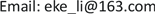1浙江师范大学，数学与计算机科学学院，浙江 金华

2金华职业技术学院，农业与生物工程学院，浙江 金华

3浙江师范大学，经济与管理学院，浙江 金华1. 引言

2. 新的无参数填充函数

( P ) { min f ( x ) , s .t .   x ∈ R n . (2.1)

{ min f ( x ) , s .t .   x ∈ X (2.2)

1) x * 是 p ( x , x * ) 的一个严格局部极大点， f ( x ) 在点 x * 处的盆谷 B * 成为 p ( x , x * ) 的峰的一部分。

2) p ( x , x * ) 在比 B * 高的盆谷里没有平稳点，即 ∇ p ( x , x * ) ≠ 0 。

3) 如果存在比 B * 低的盆谷 B 1 * ，则在 x ′ 和 x * 的连线上极小化 p ( x , x * ) 得到极小点 x ″ ∈ B * ，其中， N ( x 1 * , δ 1 ) ( δ 1 > 0 ) ， x ′ ∈ N ( x 1 * , δ 1 ) 。

1) x * ∈ B * ；

2) 对于任意一点 x ∈ B * 使得 x ≠ x * 及 f ( x ) > f ( x * ) ，存在一条从 x 到c的下降路径。

p ( x , x * ) = − ln ( 1 + ‖ x − x * ‖ 2 ) ( f ( x ) − f ( x * ) ) . (2.3)

p ( x , x * ) = − ln ( 1 + ‖ x − x * ‖ ) ( f ( x ) − f ( x * ) ) < 0 = p ( x * , x * )

f ( x ) = f ( x * ) + ( x − x * ) T ∇ f ( x ) + o ( ‖ x − x * ‖ )

f ( x ) − f ( x * ) = ( x − x * ) T ∇ f ( x ) + o ( ‖ x − x * ‖ ) > 0

∇ p ( x , x * ) = − [ 2 ( x − x * ) 1 + ‖ x − x * ‖ 2 ( f ( x ) − f ( x * ) ) + ln ( 1 + ‖ x − x * ‖ 2 ) ∇ f ( x ) ]

∇ p ( x , x * ) T ( x − x * ) = [ 2 ‖ x − x * ‖ 2 1 + ‖ x − x * ‖ 2 ( f ( x ) − f ( x * ) ) + ln ( 1 + ‖ x − x * ‖ 2 ) ∇ f ( x ) T ( x − x * ) ] < 0

p ( x , x * ) = − ln ( 1 + ‖ x − x * ‖ 2 ) ( f ( x ) − f ( x * ) ) < 0

p ( x ′ , x * ) = − ln ( 1 + ‖ x ′ − x * ‖ 2 ) ( f ( x ′ ) − f ( x * ) ) > 0

3. 填充函数算法

x * + δ ( 1 , 0 ) , x * + δ ( 0 , 1 ) , x * − δ ( 1 , 0 ) , x * − δ ( 0 , 1 )

4. 数值实验

4.1. 测试函数

1) 6-Hump Back Camel函数 

{ min f ( x ) = 4 x 1 2 − 2.1 x 1 4 + 1 3 x 1 6 − x 1 x 2 − 4 x 2 2 + 4 x 2 4 s .t .   − 3 ≤ x 1 , x 2 ≤ 3

2) Restrign函数 

{ min f ( x ) = x 1 2 + x 2 2 − cos ( 18 x 1 ) − cos ( 18 x 2 ) s .t .   − 1 ≤ x 1 , x 2 ≤ 1

3) 文献  中的一个二维函数(c = 0.05)

{ min f ( x ) = [ 1 − 2 x 2 + c * sin ( 4 π x 2 ) − x 1 ] 2 + [ x 2 − 0.5 sin ( 2 π x 1 ) ] 2 s .t .   − 10 ≤ x 1 , x 2 ≤ 10

4.2. 数值结果

k ：求解第 k 次局部极小点的迭代步数；

x k ：满足 x k ∈ X 的第 k 次初始点；

x k * ：第 k 个局部极小点；

f ( x k * ) ：第 k 个局部极小点的目标函数值；

iter ：算法迭代步数；

x * ：算法最终得到的全局极小点；

f ( x * ) ：算法最终得到的全局极小值。

Original value x = ( 1 , 2 )
kx kx k *f ( x k * )
1( 1 2 )( 1.70360672 0.79608357 )−0.21546382438372103
2( 0.96346164 0.76342669 )( 0.08984232 0.71265641 )−1.0316284534895122

Original value x = ( 1 , 1 )
kx kx k *f ( x k * )
1( 1 1 )( 1.04075871 1.04075871 )0.17977496636886192
2( 0.69788109 0.69788109 )( 8.75480363 e − 12 8.75480363 e − 12 )−2.0

c = 0.05 ，Original value x = ( 6 , − 2 )
kx kx k *f ( x k * )
1( 6 − 2 )( 5.71982142 − 1.9162353 )2.7433787691978955
2( 1.60870902 − 0.29679585 )( 1.59746304 − 0.2874076 )4.959859574394102e-15

Compared with paper [11
iterx *f ( x * )

5. 填充函数在实验数据处理中的应用5.1. 背景和问题

Reaction rate and substrate concentration data in puromycin experiment

Average the data after treatment with scorpion toxi

5.2. 分析与假设

y = f ( x , β ) = β 1 ( 1 − e − β 2 x )

min f ( x ) = ∑ i = 1 n ( y i − y ^ i ) 2 = ∑ i = 1 n ( β 1 ( 1 − e − β 2 x ​ ) − y ^ i ) 2

6. 结语